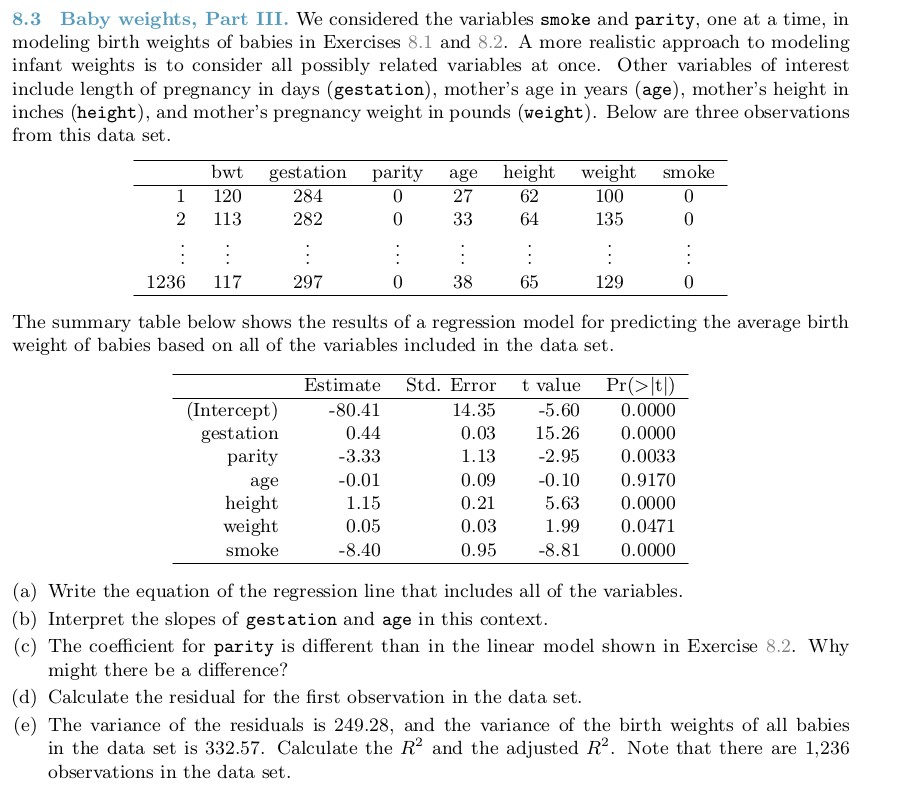#### regression line as follows:

$\hat{bwt} = -80.41 + 0.44 * \hat{gestation} - 3.33 * \hat{parity} - 0.01 * \hat{age} + 1.15 * \hat{height} + 0.05 * \hat{weight} - 8.40 * \hat{smoke}$

#### d) The residual of the first observation is calculated as follows:

$\hat{e_1} = bwt_1 - \hat{bwt_1} = 120 - (-80.41 + 0.44 * 284 - 3.33 * 0 - 0.01 * 27 + 1.15 * 62 + 0.05 * 100 - 8.40 * 0) = -0.58$

#### formulas below:

$$R^2 = 1 - (var(e_{i}) / var(y_{i}))$$ and

$$R^2_{adj} = R^2 * (n-1 / n-k-1)$$

#### In this case, n = 1236, k = 6 (6 predictors)

$$var(e_{i}) = 249.28$$ and $$var(y_{i}) = 332.57$$

#### Plugging in the numbers gives us

$$R^2 = 1 - (249.28 / 332.57) = 0.25$$ (rounded to two decimal places)

$$R^2_{adj} = 0.25 * (1235 / 1229) = 0.25$$